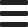# How to Get the Number of Files in a Folder with PHP

In this tutorial, I will demonstrate how to get the number of files (and subfolders) in a folder using a simple PHP script.

In another tutorial, I demonstrated how to get all files in a folder with PHP using glob() and readdir() functions. In that examples, we returned information about the files within a folder but we didn't get the file count. Continuing from where we left, we will now see how to count the number of files and subfolders in a folder.

You may need to get the file count of a folder for various purposes such as displaying that number on a page or applying a limit to the number of files that can be uploaded to a folder. As usual, there is not one single best method to do something in PHP, so you are free to use whichever you want as it fits your code, as long as it does the job properly.

Now, let's see how to get the number of files in a folder.

## Get the Number of Files in a Folder with PHP

Let's say we have a folder on our website (or web application) that is named as photos and it contains images that you uploaded to your website. Assume that there are only files (photos) and no subfolders in this photos folder.

You can use the following code using the glob() function to get the number of files:

<?php
\$photos = glob('photos/*');
\$number_of_photos = count(\$photos);
?>

Simply replace the folder name/path with yours as needed. The first line gets all the photos inside the photos folder with the help of the wildcard (*) character; note that this will get all types of files whether they are image files or not. The second line gets the number of photos using the count() function.

Similarly, you can use the readdir() function to get the file count as follows:

<?php
\$number_of_photos = 0;

if (\$tmp = opendir('photos/')) {
while ((\$photo = readdir(\$tmp)) !== false) {
if (\$photo != '.' && \$photo != '..') {
\$number_of_photos++;
}
}
}
?>

TIP: We excluded from the count the . and .. that readdir() returns for the current (photos) and parent folder.

## Get the Number of Files with a Specific File Type in a Folder with PHP

Let's say we have an images folder that contains .jpg, .png and .gif images and we want to get the count for each image type. Here is the code to use:

\$jpgs = glob('images/*.jpg');
\$pngs = glob('images/*.png');
\$gifs = glob('images/*.gif');

\$number_of_jpgs = count(\$jpgs);
\$number_of_pngs = count(\$pngs);
\$number_of_gifs = count(\$gifs);

The same example with readdir() will be as follows (for counting .jpg images):

<?php
\$number_of_jpgs = 0;

if (\$tmp = opendir('images/')) {
while ((\$image = readdir(\$tmp)) !== false) {
if (strrpos(\$image, '.jpg') == (strlen(\$image) - 4)) {
\$number_of_jpgs++;
}
}
}
?>

To filter the image files with the .jpg extension, we did an IF check to see if an image file name ends with .jpg using the strrpos() and strlen() functions.

## Get the Number of All Files & Subfolders in a Folder Recursively with PHP

The above examples assumed that you have only files within the target folder. It is possible to have multiple subfolders in a folder and multiple files within each subfolder such as an images folder with multiple categories. To get the total number of files in this case, you can use the following code:

function count_files(\$dir) {
\$count = 0;

if (\$tmp = opendir(\$dir)) {
while ((\$file = readdir(\$tmp)) !== false) {
if (\$file != '.' && \$file != '..') {
if (is_dir(\$dir.'/'.\$file)) {
\$count += count_files(\$dir.'/'.\$file);
} else {
\$count++;
}
}
}
}
return \$count;
}

\$number_of_files = count_files('images');

We needed to use a custom recursive function, count_files() in order to be able to get all the files within multiple levels of subfolders. In the same manner, if you need to get only the number of subfolders in a folder, you can use the following code:

function count_folders(\$dir) {
\$count = 0;

if (\$tmp = opendir(\$dir)) {
while ((\$file = readdir(\$tmp)) !== false) {
if (\$file != '.' && \$file != '..') {
if (is_dir(\$dir.'/'.\$file)) {
\$count += count_folders(\$dir.'/'.\$file) + 1;
}
}
}
}
return \$count;
}

\$count = count_folders('images');

We defined a custom recursive count_folders function to count all the subfolders in the images folder.